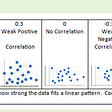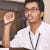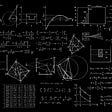# Math for Data Science — Lecture 01 Basic Matrix Operations via Python

In this lecture, we will study Matrix Operations (Initialization, Vector, Multiplication, Transpose, Special Matrix) via Python.

Matrix Definition

Matrix Notation

Matrix Initialization

`import numpy as npprint(np.zeros((2,3)))print(np.random.randint(low = 5,high=12, size=(2, 3)))A = np.matrix([[1, 2], [3, 4], [5,6]])print("Type of Matrix A:{}, having {} rows and {} columns".format(type(A),A.shape,A.shape))`

Vectors

• Row vector is having 1 row and N columns
• Column vector is having N rows and 1 column

Matrix Equality

Matrix Multiplication

In below Image 09, matrix multiplication is shown both via python code and hand as well.

• Matrix multiplication isn’t commutative (AB != BA)

Matrix Transpose

Rules of Matrix Transpose

Special Matrix

Reduce size of Sparse Matrix using CSR (Compressed Sparse Row)

Properties of Determinants

WORK IN PROGRESS !! Jupyter Notebook/.py will be uploaded soon..

Our Next lecture is about using Elementary row operations to find Row Echelon Form (REF), Reduced Row Echelon Form (RREF) and Rank of Matrix (https://medium.com/math-for-data-science/math-for-data-science-lecture-02-elementary-row-operations-via-python-46885a33a84c).

--

--

--

## More from Math for data science

Idea is to understand math for data science from intuition and code (mainly python)

## A beginner’s approach to Solve a Data Science Problem## Graph Algorithms for Risk Assessment## Predictive Modeling in Healthcare Analytics## Data Visualization Capstone Project## Correlation## Data Scientist to Product Manager? It’s not a common path, they say## TUTORIAL SERIES: 6 CHARACTERISTICS OF SUCCESSFUL TRADERS## Aakash Goel

Senior Data Scientist @ Fractal Analytics

## Exploring Singular Value Decomposition(SVD) from scratch in python## Data Science 🤝 Math (Linear algebra)## The Zeta transform: definition, theorems, examples — The Tech Goggler